Web www.handwritingforkids.com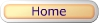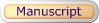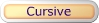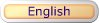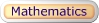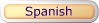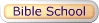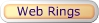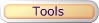### Mathematics - Division

This printable basic math division worksheet generator will provides plenty of math practice, boost test scores and prepare the child/children to meet the Grade 3-5 curriculum standards. This math worksheet maker will create a division equations with handwriting lines. No Remainders

See sample math worksheets below:

 3 5 15

This printable basic math division worksheet generator will provides plenty of math practice, boost test scores and prepare the child/children to meet the Grade 3-5 curriculum standards. This math worksheet maker will create a division equations without handwriting lines. No Remainders

See sample math worksheets below:

 3 5 15

This online/interactive math division generator will provides plenty of online math practice, boost test scores and prepare the child/children to meet the Grades 3-5 curriculum standards. This online/interactive math program, will create an online division equations with an option of timed test, and it will automatically graded/scored your results in a printable worksheet. No Remainders

Type of online math problems:

• Online Math 1 - Dividing through 18
• Online Math 1 - Dividing through 36
• Online Math 1 - Dividing through 54
• Online Math 1 - Dividing through 81
• and More!

 3 5 15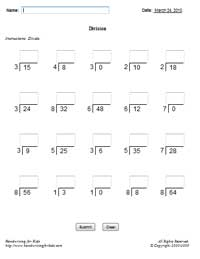This worksheet generator will create your own customized/personalized division worksheet. You enter your own math problems in a printable worksheet. Maximum 5 digits x 5 digits (5x5).

 31 5 155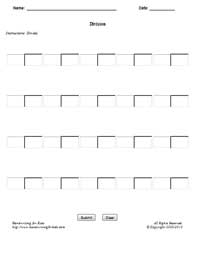This printable basic math division worksheet generator will provides plenty of math practice, boost test scores and prepare the child/children to meet the Grade 3-5 curriculum standards. This math worksheet maker will create a horizontal division equations with handwriting lines. No Remainders

See sample math worksheets below:

 15 ÷ 5 = 3

This printable basic math division worksheet generator will provides plenty of math practice, boost test scores and prepare the child/children to meet the Grade 3-5 curriculum standards. This math worksheet maker will create a horizontal division equations with handwriting lines. No Remainders

See sample math worksheets below:

 4 ÷ 2 = 2

This online/interactive math division generator will provides plenty of online math practice, boost test scores and prepare the child/children to meet the Grades 3-5 curriculum standards. This online/interactive math program, will create an online horizontal division equations with an option of timed test, and it will automatically graded/scored your results in a printable worksheet. No Remainders

Type of online math problems:

• Online Math 2 - Dividing through 18
• Online Math 2 - Dividing through 36
• Online Math 2 - Dividing through 54
• Online Math 2 - Dividing through 81
• and More!

 12 ÷ 4 = 3### Division - Tables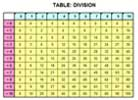Examples Division Tables How to use the division table Blank Table #1 Answer SheetDivision 0-12 Flash Cards (MS Word Document - Printable Only) Flash Cards Printing Instructions: 1. Print all odd pages 2. Flip the stack of printed pages and re-insert into the printer. 3. Print all even pages. Suggestion: Print on color paper or card-stock paper.

Math: Animation - How to Do Long Division

### JavaScript Interactive Math* - Division

 JavaScript Math (Interactive)*

* JavaScript must be enabled on your browser.

Daily Sudoku for Kids (Picture Sudoku) 4x4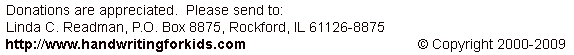Basic Handwriting for Kids Free for Non-Profit Use Ms. Readman, Rockford, IL Revised: